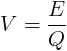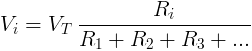# Electrical voltage

## Voltage definition

Voltage, electric potential difference, electromotive force (emf), electric pressure or electric tension is the difference in electric potential between two points, which (in a static electric field) is defined as the work needed per unit of charge to move a test charge between the two points. In the International System of Units, the derived unit for voltage (potential difference) is named volt. In SI units, work per unit charge is expressed as joules per coulomb, where 1 volt = 1 joule (of work) per 1 coulomb (of charge). The old SI definition for volt used power and current; starting in 1990, the quantum Hall and Josephson effect were used, and recently (2019) fundamental physical constants have been introduced for the definition of all SI units and derived units. Voltage or electric potential difference is denoted symbolically by ∆V, simplified V, or U, for instance in the context of Ohm's or Kirchhoff's circuit laws.

Electric potential differences between points can be caused physically by electric charge build up or imbalance (eg. well known "static" and electronic capacitor) also by electric current through a magnetic field, and by time-varying magnetic fields (eg. dynamo or generator), or some combination of these three. Additionally on a macroscopic scale potential difference can be caused by electrochemical processes (cells and batteries) and pressure induced piezoelectric effect and heat induced emf across metal junctions. These latter processes at microscopic level have the physical origins previously mentioned. A voltmeter can be used to measure the voltage (or potential difference) between two points in a system; often a common reference potential such as the ground of the system is used as one of the points. A voltage may represent either a source of energy (electromotive force) or lost, used, or stored energy (potential drop).

Using water pipe analogy, we can visualize the voltage as height difference that makes the water flow down.

V = φ2 - φ1

V is the voltage between point 2 and 1 in volts (V).

φ2 is the electric potential at point #2 in volts (V).

φ1 is the electric potential at point #1 in volts (V).

In an electrical circuit, the electrical voltage V in volts (V) is equal to the energy consumption E in joules (J)

divided by the electric charge Q in coulombs (C).V is the voltage measured in volts (V)

E is the energy measured in joules (J)

Q is the electric charge measured in coulombs (C)

## Voltage in series

The total voltage of several voltage sources or voltage drops in series is their sum.

VT = V1 + V2 + V3 +...

VT - the equivalent voltage source or voltage drop in volts (V).

V1 - voltage source or voltage drop in volts (V).

V2 - voltage source or voltage drop in volts (V).

V3 - voltage source or voltage drop in volts (V).

## Voltage in parallel

Voltage sources or voltage drops in parallel have equal voltage.

VT = V1 = V2 = V3 =...

VT - the equivalent voltage source or voltage drop in volts (V).

V1 - voltage source or voltage drop in volts (V).

V2 - voltage source or voltage drop in volts (V).

V3 - voltage source or voltage drop in volts (V).

## Voltage divider

For electrical circuit with resistors (or other impedance) in series, the voltage drop Vi on resistor Ri is:## Kirchhoff's voltage law (KVL)

The sum of voltage drops at a current loop is zero.

Vk = 0

## DC circuit

Direct current (DC) is generated by a constant voltage source like a battery or DC voltage source.

The voltage drop on a resistor can be calculated from the resistor's resistance and the resistor's current, using Ohm's law:

##### Voltage calculation with Ohm's law

VR = IR × R

VR - voltage drop on the resistor measured in volts (V)

IR - current flow through the resistor measured in amperes (A)

R - resistance of the resistor measured in ohms (Ω)

## AC circuit

Alternating current is generated by a sinusoidal voltage source.

##### Ohm's law

VZ = IZ × Z

VZ - voltage drop on the load measured in volts (V)

IZ - current flow through the load measured in amperes (A)

Z - impedance of the load measured in ohms (Ω)

##### Momentary voltage

v(t) = Vmax × sin(ωt)

v(t) - voltage at time t, measured in volts (V).

Vmax - maximal voltage (=amplitude of sine), measured in volts (V).

t        - time, measured in seconds (s).

##### RMS (effective) voltage

VrmsVeff  =  Vmax / √2 ≈ 0.707 Vmax

Vrms -  RMS voltage, measured in volts (V).

Vmax - maximal voltage (=amplitude of sine), measured in volts (V).

Vp-p = 2Vmax

## Voltage drop

Voltage drop is the drop of electrical potential or potential difference on the load in an electrical circuit.

## Voltage Measurement

Electrical voltage is measured with Voltmeter. The Voltmeter is connected in parallel to the measured component or circuit.

The voltmeter has very high resistance, so it almost does not affect the measured circuit.

## Voltage by Country

AC voltage supply may vary for each country.

European countries use 230V while north America countries use 120V.

Country Voltage
[Volts]
Frequency
[Hertz]
Australia 230V 50Hz
Brazil 110V 60Hz
China 220V 50Hz
France 230V 50Hz
Germany 230V 50Hz
India 230V 50Hz
Ireland 230V 50Hz
Israel 230V 50Hz
Italy 230V 50Hz
Japan 100V 50/60Hz
New Zealand 230V 50Hz
Philippines 220V 60Hz
Russia 220V 50Hz
South Africa 220V 50Hz
Thailand 220V 50Hz
UK 230V 50Hz
USA 120V 60Hz
###### source: Wikipedia

Electrical Current »

Currently, we have around 5612 calculators, conversion tables and usefull online tools and software features for students, teaching and teachers, designers and simply for everyone.